Select Page

# Leftmost Derivation in Automata

Getting a string by expanding the leftmost non terminal at each step is called as leftmost derivation in Automata.

• The representation of leftmost derivation in the form of tree is called as a leftmost derivation tree.
• In the leftmost derivation tree, we read the given input string (W) from left to right.

## Example of Leftmost Derivation

Consider the following production rules for the grammar (G)

S → xB / yA

S → xS / yAA / x

B → yS / xBB / y

Consider a string W = xxxyyxyyyx

Let us derive the given string (W= xxxyyxyyyx) by using given production rules of grammar. And will also draw the derivation tree of each step as given below

## Leftmost Derivation and Tree

Let us see derivation through production rules and their tree as given below

Step 01: S   → xB                    (W=xB)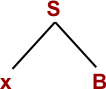Step 02: W= xxBB                   (Using B → xBB)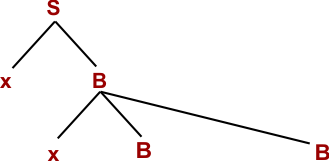Step 03: W= xxxBBB                (Using B → xBB)Step 04: W= xxxyBB                (Using B → y)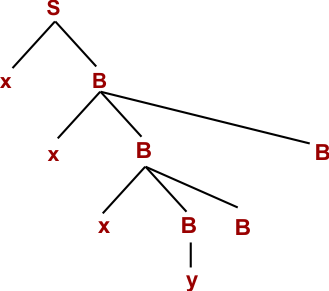Step 05: W= xxxyyB                (Using B → y)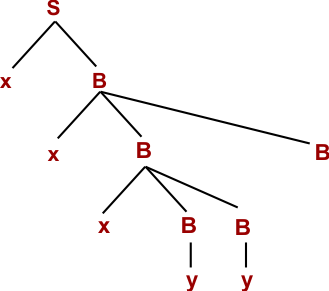Step 06: W= xxxyyxBB            (Using B → xBB)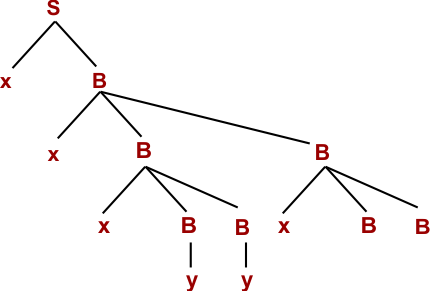Step 07: W= xxxyyxyB            (Using B → y)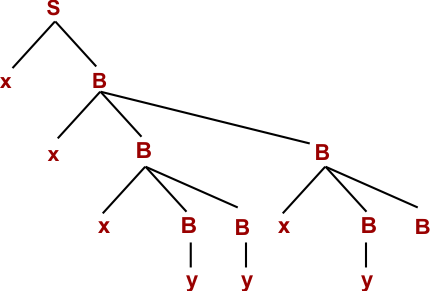Step 08: W= xxxyyxyyS          (Using B → yS)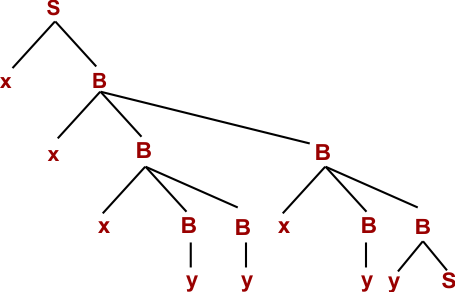Step 09: W= xxxyyxyyyA        (Using S → yA)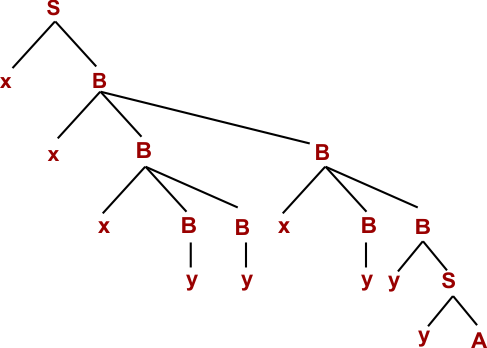Step 10: W= xxxyyxyyyx        (Using A → x)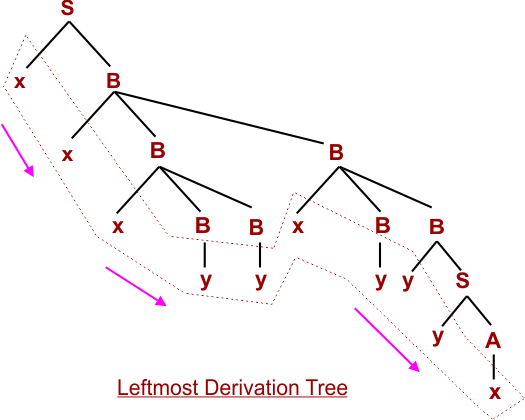Hence proved the given string (W= xxxyyxyyyx) is derived through leftmost derivation. So, given string belongs to Given Grammar (G).

Help Other’s By Sharing…